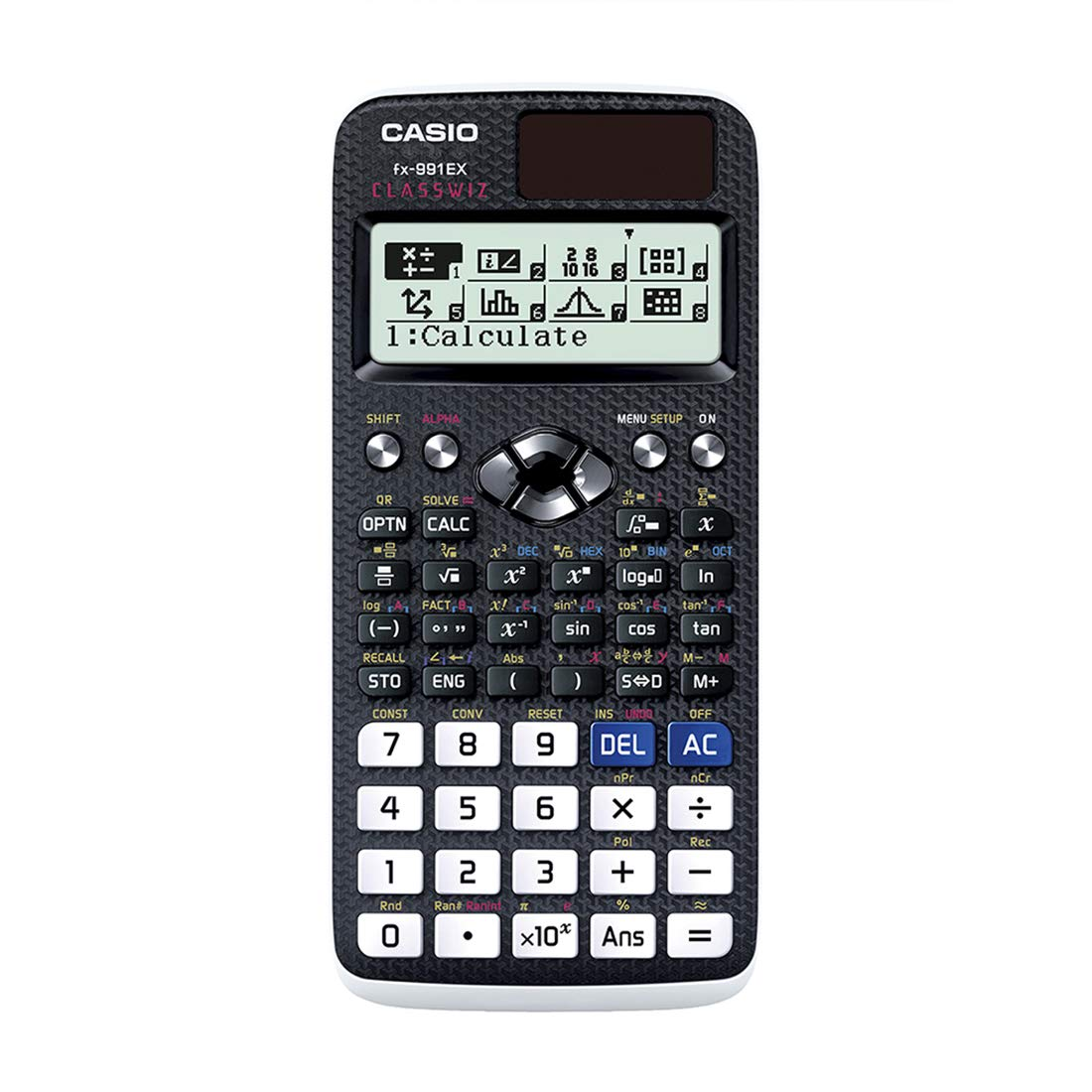•••# Casio Calculator (FX991EX)

Regular price
Rs 5,700.00 LKR
Regular price
Sale price
Rs 5,700.00 LKR

•GCSE/AS/A LevelSuitable for Cambridge International Examinations GCSE, AS & A Level.

•Number of Functions : 552

•Natural textbook displayDisplay expression same as textbook.

•10 + 2 digits10-digit mantissa + 2-digit exponential display.

--

### Intuitive, easy-to-learn icon displayThe use of icons on the menu screen improves viewability. Selection of the desired function is quick and easy.

### Natural Textbook DisplayInput and display fractions, powers, logarithms, roots, and other mathematical formulas and symbols just as they appear in textbooks.

### List display function for thorough, speedy learningVariables and statistical calculation results stored in memory can be displayed in lists. There’s no need to recall and confirm individual values as with previous models.

### Easy-to-understand English messages and interactive formatNearly all English words are displayed on the screen in unabbreviated form. Interactive menu display realizes more intuitive operation.

### High computational competence for performing even advanced mathematicsClassWiz contains calculation functions that support even advanced mathematical operations, including spreadsheet calculations, 4 × 4 matrix calculations, calculation of simultaneous equations with four unknowns and quartic equations, and advanced statistical distribution calculations.